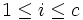# Difference between revisions of "Supersolvable implies nilpotent derived subgroup"

This article gives the statement and possibly, proof, of an implication relation between two group properties. That is, it states that every group satisfying the first group property (i.e., supersolvable group) must also satisfy the second group property (i.e., group with nilpotent derived subgroup)
View all group property implications | View all group property non-implications
Get more facts about supersolvable group|Get more facts about group with nilpotent derived subgroup

## Statement

The Derived subgroup (?) of a Supersolvable group (?) is a Nilpotent group (?). Moreover, the Nilpotence class (?) of the commutator subgroup is bounded from above by the length of any normal series for the whole group with cyclic quotients.

## Facts used

1. Commutator subgroup centralizes cyclic normal subgroup
2. Commutator subgroup is normal
3. Normality is strongly intersection-closed
4. Normality satisfies image condition
5. Second isomorphism theorem
6. Cyclicity is subgroup-closed
7. Commutator subgroup satisfies image condition: Under a surjective homomorphism, the image of the commutator subgorup equals the commutator subgroup of the image.

## Proof

Given: A supersolvable group$G$ with a normal series$1 = N_0 \le N_1 \le \dots N_c = G$ with$N_i/N_{i-1}$ cyclic.

To prove:$[G,G]$ is nilpotent of class at most$c$.

Proof: We will prove that the series:$1 = N_0 \cap [G,G] \le N_1 \cap [G,G] \le \dots \le N_c \cap [G,G] = [G,G]$

is a central series for$[G,G]$.

1.$[G,G]$ is normal in$G$: This is fact (2).
2.$N_i \cap [G,G]$ is normal in$G$ for all$i$: This follows from fact (3).
3.$(N_i \cap [G,G])/(N_{i-1} \cap [G,G])$ is normal in$G/(N_{i-1} \cap [G,G])$ for all$1 \le i \le c$: This follows from fact (4).
4.$(N_i \cap [G,G])/(N_{i-1} \cap [G,G])$ is cyclic: By the second isomorphism theorem (fact (5)), this quotient is isomorphic to$N_{i-1}(N_i \cap [G,G])/N_{i-1}$, which in turn is a subgroup of$N_i/N_{i-1}$. Fact (6) therefore yields that it is cyclic.
5.$[G,G]/(N_{i-1} \cap [G,G])$ is the commutator subgroup of$G/(N_{i-1} \cap [G,G])$: This is direct from fact (7).
6.$(N_i \cap [G,G])/(N_{i-1} \cap [G,G])$ is in the center of$[G,G]/(N_{i-1} \cap [G,G])$: By the previous three steps,$)N_i \cap [G,G])/(N_{i-1} \cap [G,G])$ is cyclic normal in$G/(N_{i-1} \cap [G,G])$, and$[G,G]/(N_{i-1} \cap [G,G])$ is the derived subgroup. So fact (1) yields that$(N_i \cap [G,G])/(N_{i-1} \cap [G,G])$ is in the center of$[G,G]/(N_{i-1} \cap [G,G])$.

Thus, the series is indeed a central series.